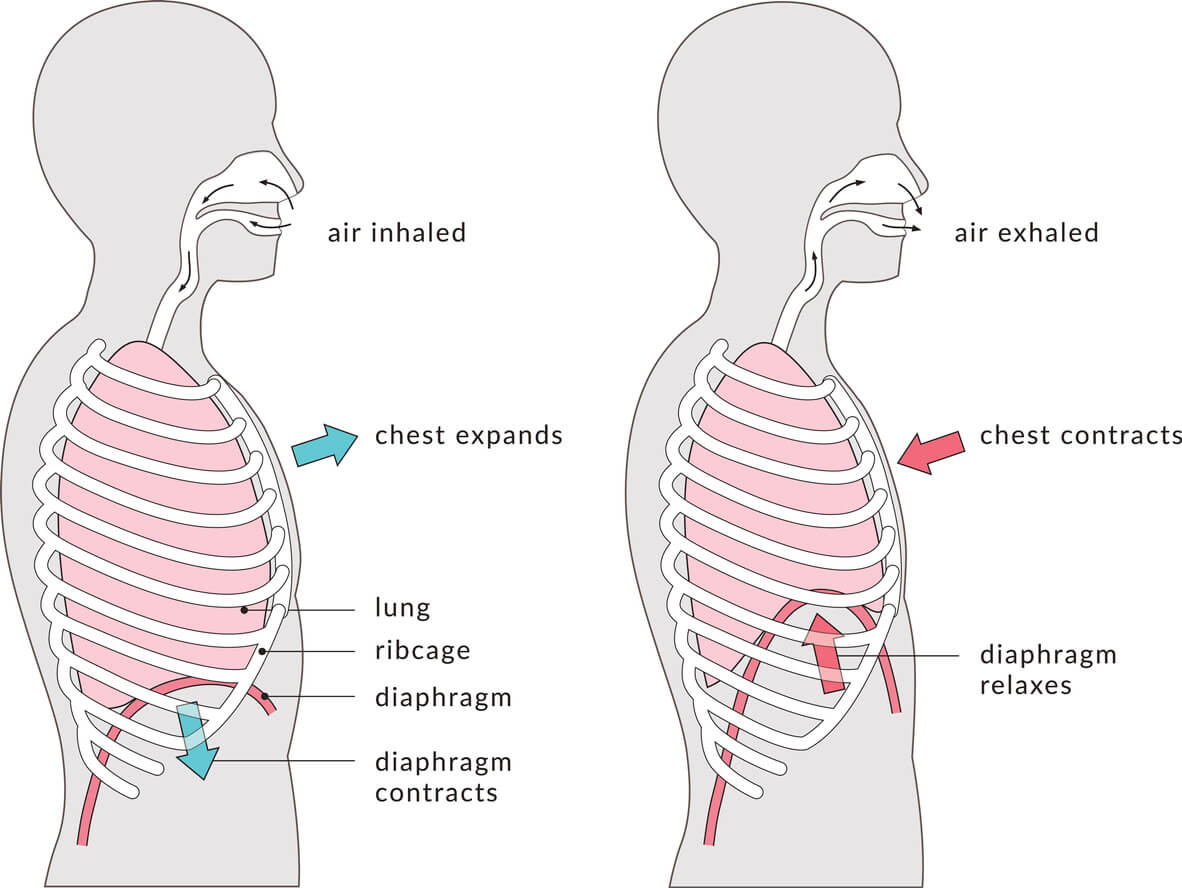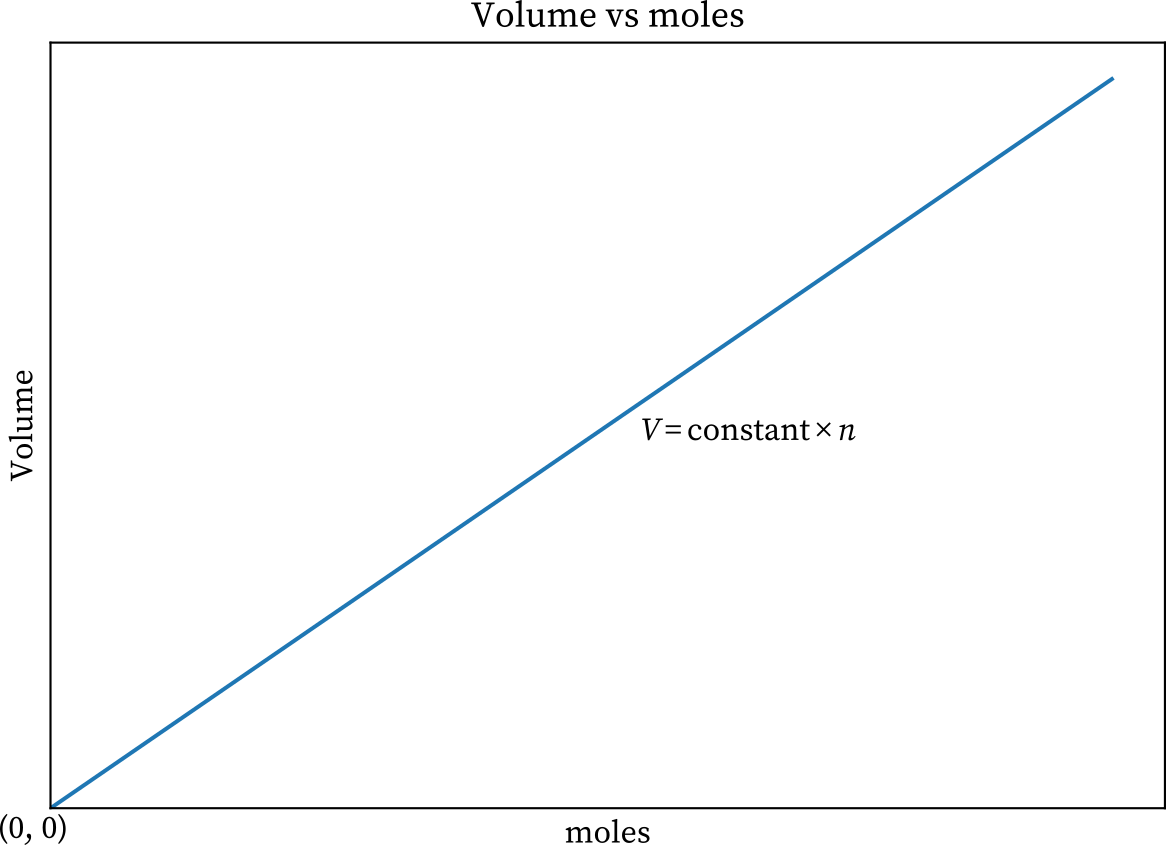Sarah Taylor

HS-PS1-3

Table of content

### Lesson Objectives:

• Describe the relationship between the amount of gas, n, and the volume, V, of its container at a constant pressure and temperature
• Solve problems using Avogadro’s equation
• Derive Avogadro’s law from the ideal gas law

## IntroductionSource

Did you know that every time you breathe in you’re demonstrating Avogadro’s law in action? The law states that the total number of atoms or molecules of any gas is directly proportional to the gaseous volume occupied at constant pressure and temperature. In basic terms, when the number of gas particles increases, the volume of the space the gas occupies increases proportionally.

When you take a breath in, you’re breathing in gas particles from the air around you. The addition of these gas particles increases the volume of your lungs as they expand. This expansion keeps pressure constant and the temperature of the air remains the same as well. Thus, as per Avogadro’s law, an increase in particle concentration leads to an increase in volume when pressure and temperature are held constant.

The law is named after Italian scientist Amedeo Carlo Avogadro, who conveyed that two different ideal gasses occupying the same volume at a given pressure and temperature will contain the same number of atoms or molecules.

Avogadro’s Law states that the total number of atoms or molecules of any gas is directly proportional to the gaseous volume occupied at constant pressure and temperature.

Avogadro’s law is linked with the ideal gas equation since it connects factors like pressure, volume, temperature, and amount of substance for the given gas.

### Avogadro’s Law Formula & Derivation

For a given mass of ideal gas, the total number of atoms or molecules of any gas is directly proportional to the gaseous volume occupied at constant pressure and temperature. The mathematical representation of Avogadro’s law is:

V = k ྾ n or V1/n1 = V2/n2

Where:

• V is volume
• k is a constant
• n is the number of gas particles in moles.

Avogadro’s law can be easily derived using the ideal gas equation:

PV = nRT

Where:

• P is the pressure exerted by the gas on the container wall
• V is the gaseous volume
• n is the amount of gas in moles
• R is the universal gas constant
• T is the absolute temperature of the gas

Because Avogadro’s law states that temperature and pressure remain constant, we can replace them with the constant k to translate it into the algebraic expression:

V/n = k

When we rearrange this equation, we find:

V = k ྾ n

From this equation we can see that as the number of gas particles increases, so does the volume. Let’s try out a practice problem to see how it works.Source

At a constant pressure and temperature, 10.0 L of a gas contains 1.125 moles. If the amount of gas is increased to 1.342, what new volume will result?

Step 1: Define the variables

• V1 = 10.0 L
• V2 = ?
• n1 = 1.125 mol
• n2 = 1.342 mol

Step 2: Choose an equation

For the purpose of this calculation, let’s use the equation format V1/n1 = V2/n2.

Step 3: Calculate

V1 ÷ n1 = V2 ÷ n2 V1 × n2 = V2 × n1 10.0 L × 1.342 mol = V2 × 1.125 mol V2 = (10.0 L × 1.342 mol)/1.125 mol V2 = 11.929 L

The new volume of the gas is 11.929 L.

## Molar Volume

As Avogadro’s Law states, the ratio of the gaseous substance amount and the volume remains constant when the values of pressure and temperature are constant, and this ratio is denoted by k. Hence,

K = (RT)/P

Under standard pressure and temperature T= 273.15k and P= 101.325 kiloPascals Thus,

The volume of given one-mole gas at STP

= (8.315 J.mol-1.K-1)(273.15k)/(101.325kPa)

=22.4 liters

## 💡 Summary

• Avogadro’s law states that the total number of atoms or molecules of any gas is directly proportional to the gaseous volume occupied at constant pressure and temperature.
• Avogardro’s equation is written as V = k ྾ n or V1/n1 = V2/n2.

## 🧐 FAQs

1. What is Avogadro’s gas law formula?

Avogadro’s Law specifies that the total number of molecules or atoms of gas remains directly proportional to the volume that the gas occupies at constant pressure and temperature.

2. What Is Avogadro’s law class 11?

Avogadro’s Law states that equal volumes of all gasses, at the same temperature and pressure, have the same number of molecules.

We hope you enjoyed studying this lesson and learned something cool about Avogadro’s Law! Join our Discord community to get any questions you may have answered and to engage with other students just like you! Don't forget to download our App to experience our fun VR classrooms - we promise, it makes studying much more fun! 😎